##1. Homographic Relation

Homographic Relation between two real variables x, y is a one-to-one (invertible) function y=F(x) involving algebraic operations only between the variables x, y. The one-to-one and the algebraic requirements imply that the relation has the form.
y = (ax + b)/(cx + d),       with non zero determinant ad-bc (*).
Equivalently.
cxy-ax+dy-b=0                  (**).
Hence the graph of the function is a rectangular hyperbola. This is the only kind of quadratic curve that represents an invertible real function of the extended line to itself.
By extended line I mean a line where an additional point (at infinity) has been added.
The main properties of a homographic relation are.
 They preserve the cross ratio  (x1,x2,x3,x4) = [(x3-x1)/(x3-x2)]:[(x4-x1)/(x4-x2)] of four points.
This means that the corresponding cross ratio (y1,y2,y3,y4) = (x1,x2,x3,x4).
 They are distinguished in involutive (i.e. such that F2=1) and non-involutive.
  Involutive/non-involutive homographic relations are completely determined by prescribing arbitrarily the values to two/three arbitrary points.
 If a homographic relation f interchanges two points, i.e. there are (x,y) such that f(x)=y and f(y)=x, then f is involutive.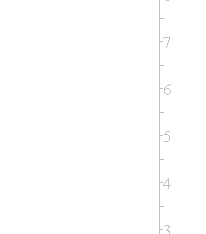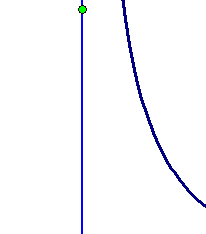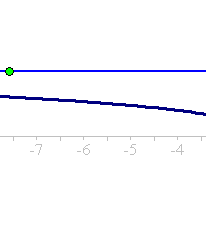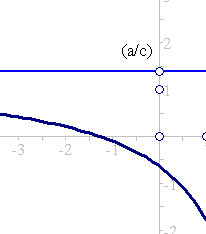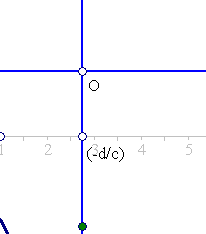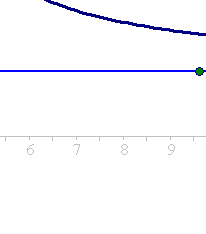1]  The first is an easy calculation.
2]  Non-involutive homographies are determined by their values at three distinguished points.
This follows from () the equality of cross ratios  (x1,x2,x3,x) = (y1,y2,y3,y), which solved for y determines the homography.
3+4] The involutive property amounts to the symmetry of the hyperbola about the first diagonal (line y=x).
This in turn is equivalent with the condition.
a+d = 0.
This is equivalent also to the lying of the center O of the hyperbola on the first diagonal.
Involutive homographies are determined by their values at two points. This is seen most easily from (**). The homographic relation obtains in this case the form.
Axy+B(x+y)+C=0  (***).
This determines the coefficients {A,B,C} (up to a multiple) uniquely by giving two pairs (x1,y1), (x2,y2).
General examples of homographies can be easily constructed by defining one-to-one maps y = F(x) of the points of a line L to the points of a  line L' involving geometric constructions with intersection points.
a)  of two lines.
b) of a line and a conic.
Such constructions reduce to algebraic relations of (x,y), thus defining homographic relations.
An example of a homographic relation defined geometrically is given in RectHypeRelation.html .

##2. Fixed points of homographic relations

Of particular interest are the fixed points of such relations, characterized by the equation x = F(x) = (ax+b)/(cx+d). This is equivalent to the quadratic equation cx2 +(d-a)x -b = 0, with discriminant D = (a+d)2-4(ad-bc). Involutive relations (a+d=0) have either no (when ad-bc > 0) or two (when ad-bc < 0) fixed points. This follows from the derivative F'(x)=(ad-bc)/(cx+d)2. Only hyperbolas representing decreasing functions ((ad-bc)<0) meet the first diagonal. This property of involutions is used in the file FourPtsAndTangent.html .
Assume F is an involution and there are two different, real or imaginary fixed points x1, x2. Then, since F preserves the cross ratio, for any other point x3, x4=F(x3) we have (x1,x2,x3,x4) = (F(x1),F(x2),F(x3),F(x4)) = (x1,x2,x4,x3). This impies (x4-x1)/(x4-x2) = -(x3-x1)/(x3-x2) i.e. (x3, x4=F(x3)) are harmonic conjugate with respect to (x1,x2). Thus, involutions are characterized by the existence of two (real or imaginary) points x1, x2, such that for every other point x3 the image x4=F(x3) is the harmonic conjugate of x3 with respect to x1, x2.

##3. Homographic relations as projectivities

The importance of this kind of relations stems from the fact that they represent projectivities (or homographies) of one-dimensional projective spaces or projective lines (see ProjectiveLine.html ). The standard model of such a line is the one-point compactified real line consisting of the real numbers to which a point at infinity has been added.
Another model of the projective line is a range of points consisting of points of a line (in the plane) to which a point at infinity has been added. A third type of projective line is the pencil of lines (of the plane) emanating from a fixed point, sometimes called a range of lines. In all these models their points can be described by classes of pairs of numbers (x,y) different from (0,0), two pairs considered in the same class if they are related by (x',y')=k(x,y), with a non-zero k. A projectivity or homography is described in these coordinates by an invertible matrix: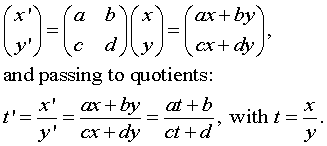##4. Orthogonality for pencils in involution

Next example handles a question in a typical example of a pencil of lines. Namely the lines emanating from the origin of coordinates. Let a line through the origin intersect the line x=1 at a point (1,m). This line is represented by the equation y=mx, and (1,m) are projective coordinates parametrizing the pencil of lines through the origin. By the previous discussion an involution of this pencil is defined through a function m'=F(m), with F(m)=(am+b)/(cm+d). By the involution condition d must be equal to -a.
Problem To find the pairs of lines (m, m'=F(m)) which are orthogonal.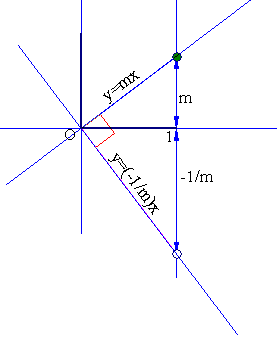If the line corresponding to m'=F(m) is orthogonal to the one corresponding to m, m' must be equal to -1/m. Thus to locate pairs (m,m') which are orthogonal we must solve the equation -(1/m)=(am+b)/(cm-a), which is equivalent to am2+(b+c)m-a=0.
 If a is non-zero, setting (b+c)/a = 2d, we arive at equation m2+2dm-1=0, wich has always two real solutions m1, m2. Since their product m1m2=-1, the two solutions determine the same pair of orthogonals.
 If  a is zero, then the equation above becomes (b+c)m=0 i.e. b+c=0, and F(m)=b/(cm)=-1/m. Thus, in this case we have the special involution by orhtogonals, in which every line maps to its orthogonal.
The result of this short discussion is: An involution on a pencil of lines either has exactly one pair (m,m') of orthogonal lines or all pairs (m,m') are orthogonal.

##5. Involutions and circle bundles

The fixed points of a general homographic relation are given by the equation x = F(x) = (ax+b)/(cx+d), equivalent to the quadratic equation cx2 +(d-a)x -b = 0, with discriminant.
- if D>0, then there are two real fixed points and the relation is called hyperbolic.
- if D<0, then there are two imaginary fixed points and the relation is called elliptic.
- if D=0, then there are two real coincident fixed points and the relation is called parabolic.
Denoting by {x1,x2} the two fixed points, preservation of cross ratio implies (x1,x2,x,x')=(x1,x2,x',x) (putting x'=F(x)).
This cross ratio is easily calculated.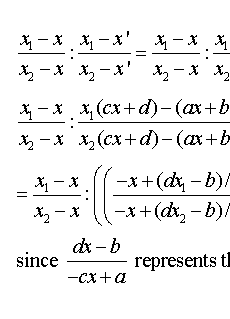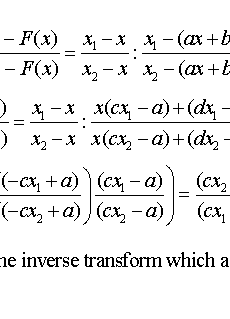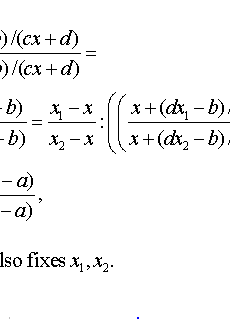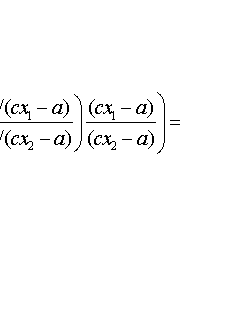Last expression is constant, equal to k say. Thus,
the general homographic relation is characterized by this constant k and the property    (x1,x2,x,x')=k,     for all pairs of points (x,x'=F(x)).
The particular case of involutions corresponds to the constant k=-1.
Inversely, the previous equation, solving for x', implies the homographic relation: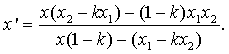Note that for x = (1/(1-k))(x1 - kx2), having ratio (x-x1)/(x-x2) = k, x' becomes infinite.
In the special case of involutions equation (x1,x2,x,x')=-1 is equivalent to: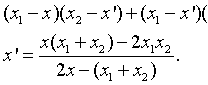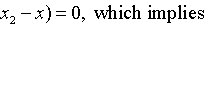Selecting the middle O with line-coordinate (x1+x2)/2 as origin, this equation becomes.
x' = r2/x, where r = (x1-x2)/2.
This means that the homography coincides with the restriction of an inversion I(O,r) on its supporting line.
From this follows that points (x,x') are intersection points of the supporting line with the members of a circle-bundle. The circle bundle being hyperbolic (ad-bc<0), elliptic (ad-bc>0) or parabolic (ad-bc=0), which is in accordance with the naming conventions at the beginning of the paragraph.

Chasles_Steiner.html
Chasles_Steiner_Envelope.html
FourPtsAndTangent.html
GoodParametrization.html
HomographicRelationExample.html
Involution.html
Line_Homography.html
ProjectiveLine.html
RectHypeRelation.html

### References

Emch Arnold Projective Geometry and its Applications John Wiley & sons, London 1905, pp. 5-14
Schwerdtfeger, Hans Geometry of complex numbers New York, Dover, 1979.